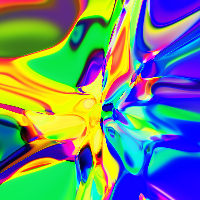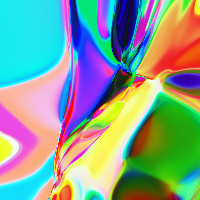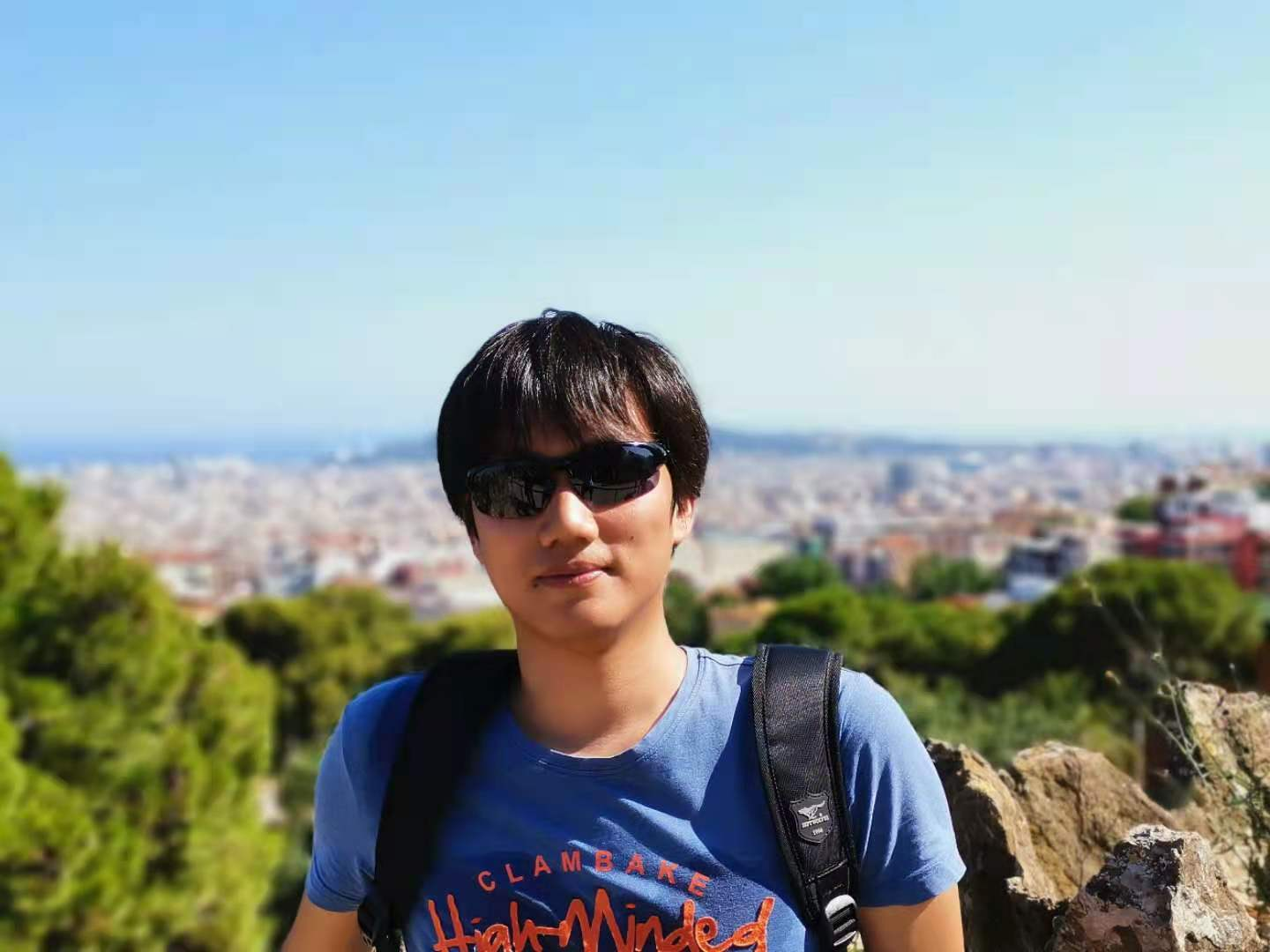# 深度强化学习中的探索策略

562

## 经典的探索策略

• $\epsilon$-贪婪算法：智能体以概率$\epsilon$随机进行探索，以$1-\epsilon$概率采用最佳动作
• 上信心界算法（Upper confidence bounds）：智能体采用可以最大化上信心界$\hat{Q}_t(a) + \hat{U}_t(a)$的动作。其中$\hat{Q}_t(a)$为与时刻$t$前动作$a$相关的平均收益；$\hat{U}_t(a)$为与动作$a$被执行次数逆相关的函数（参考这里
• 玻尔兹曼探索：智能体的动作服从玻尔兹曼分布（Q值经过softmax得到分布，使用了一个温度参数$\tau$
• 汤普森采样：智能体维护一个最优动作的概率分布，从此分布中采样动作（参考这里

• 在损失函数中加入熵：鼓励智能体采用更加多样化的动作
• 基于噪声的探索：在观察到的状态（observation）、动作甚至参数空间中加入噪声来让动作多样化

## 探索中的核心问题

### 嘈杂电视问题

“嘈杂电视”问题始于论文Burda 等人, 2018中的一个思维实验。想象一下一个强化学习智能体，它会因为不断得到新的体验而获得奖励。那么，它在面对一个不可控的、会不断显示随机画面的电视（嘈杂电视）时，它会被电视上不断出现的画面所吸引。虽然电视上的这些画面可以不断地给智能体带来了新的体验（正反馈），但是智能体本身却没有学习到任何有价值的东西，它仅仅是成为了一个“电视迷”。

## 使用内在奖励作为探索红利

• $r_t^e$为时刻$t$环境给出的外在奖励
• $r_t^i$为时刻$t$内在探索红利

1. 基于新状态的发现
2. 基于智能体关于环境认识的改善

### 基于计数的探索

$N_n(s)$为记录状态$s$访问次数（从$s_1$$s_n）的经验函数。不幸的是，实现这样一个计数函数是不现实的，因为对于绝大多数状态s$$N_n(s)=0$，这个问题在连续以及高维状态空间中尤为突出。我们希望对于绝大多数状态，$N_n(s)$可以返回一个非零值（即使是之前尚未出现过的状态）。

#### 使用密度模型

Bellemare 等, 2016使用了一个密度模型来近似状态的访问频率。他们同时设计了一个新的算法来从密度模型中推断出状态的访问次数。我们首先在状态空间上定义一个条件概率$\rho_n(s) = \rho(s \vert s_{1:n})$，它表示在给定状态$s_{1:n}$的情况下$s_{n+1}$出现的概率。对于此概率的估值，我们可以简单使用估计$N_n(s)/n$

$$\rho_n(s) = \frac{\hat{N}_n(s)}{\hat{n}} \leq \rho'_n(s) = \frac{\hat{N}_n(s) + 1}{\hat{n} + 1}$$

$\rho_n(x)$$\rho^\prime_n(x)之间的这种关系要求密度模型必须是正向学习（learning-positive）的：对于任意状态s_{1:n}\in \mathcal{S}^n以及任意s \in \mathcal{S}，有\rho_n(s) \leq \rho'_n(s)。换句话说，在观察到一个状态s出现之后，密度模型对于同一状态的预测的概率应该是增加的。除了正向学习这个属性之外，密度模型必能能够以一种在线式非随机的小批次样本的方式进行训练。这样自然而然地，我们可以有\rho'_n = \rho_{n+1} 从上面给出的线性系统，我们可以计算出伪计数：$$ \hat{N}_n(s) = \hat{n} \rho_n(s) = \frac{\rho_n(s)(1 - \rho'_n(s))}{\rho'_n(s) - \rho_n(s)} $$或者我们可以根据预测收益（prediction gain）来估计：$$ \hat{N}_n(s) \approx (e^{\text{PG}_n(s)} - 1)^{-1} = (e^{\log \rho'_n(s) - \log \rho(s)} - 1)^{-1} $$内在红利的一个常见的选择是使用r^i_t = N(s_t, a_t)^{-1/2}（类似MBIE-EB,Strehl & Littman, 2008）。基于伪计数的探索红利形式类似：r^i_t = \big(\hat{N}_n(s_t, a_t) + 0.01 \big)^{-1/2} Bellemare等,2016的实验中使用了一个简单的CTS（Context Tree Switching）密度模型来估计伪计数。CTS模型的输入为一张二维图片，输出一个基于位置的L型滤波器预测的乘积作为概率。其中每个滤波器的预测由在过去图片上进行训练的CTS算法给出。CTS算法非常简单，但是它在表达能力、可扩展性以及数据效率方面都有限制性。在之后论文中，Georg Ostrovski等,2017对此方法进行了改善，他们训练了一个PixelCNN（van den Oord 等,2016）模型作为密度模型。 正如在Zhao & Tresp，2018论文中展现的那样，密度模型也可以是高斯混合模型。在论文中，他们用了一个变分GMM来估计轨迹（连续的状态序列）的概率密度。此概率被用来在off-policy设定下作为经验回放（experience replay）的优先级。 #### 哈希后计数 另外一种让对于高维状态进行计数成为可能的想法是将状态映射为一个哈希码。这样状态出现的次数就可以被跟踪计数（Tang等, 2017）。状态空间可以一个哈希函数\phi: \mathcal{S} \mapsto \mathbb{Z}^k进行离散化。此时，探索红利定义为r^{i}(s) = {N(\phi(s))}^{-1/2}，其中N(\phi(s))$$\phi(s)$的经验计数。

Tang等，2017中提出使用一个本地敏感哈希LSH（Locality-Sensitive Hashing）来将连续、高维的数据转化为一个离散的哈希码。LSH是一类基于特定相似度量的哈希函数。如果一个哈希方案$x \mapsto h(x)$可以保留数据之间的距离信息（也就是距离相近的向量之间它们对应的哈希代码之间也比较相似），那么我们称此哈希方案为本地敏感的（如果对此感兴趣可以看看LSH在Transformer改进中是如何应用的）。SimHash是一类计算高效的LSH，它使用角度距离来衡量相似度：

$$\phi(s) = \text{sgn}(A g(s)) \in \{-1, 1\}^k$$

$$\mathcal{L}(\{s_n\}_{n=1}^N) = \underbrace{-\frac{1}{N} \sum_{n=1}^N \log p(s_n)}_\text{重建损失} + \underbrace{\frac{1}{N} \frac{\lambda}{K} \sum_{n=1}^N\sum_{i=1}^k \min \big \{ (1-b_i(s_n))^2, b_i(s_n)^2 \big\}}_\text{sigmoid 激活损失}$$

### 基于预测的探索

#### 前向动态（Forward Dynamics）

IAC依赖于一个记忆空间以及一个前向动态模型$f$。记忆空间用于存储机器人的所有经历$M=\{(s_t, a_t, s_{t+1})\}$。IAC根据状态转移逐步将状态空间（也就是机器人的感觉运动空间）分割成不同的区域。这个过程类似于决策树的构建：区域的分割发生在样本数量高于某一个阈值的时候，每一个叶子结点上，状态的多样性应该保持在一个最小值。每一个树节点都有其特色，并且有它自己的前向动态预测器$f$（称之为“专家”）。

Stadie等, 2015在一个由函数$f_\phi: (\phi(s_t), a_t) \mapsto \phi(s_{t+1})$定义的状态编码空间上训练了一个前向动态模型。模型在时刻$T$的预测误差被进行了归一化（使用截止时间$t$的所有误差的最大值进行归一化$\bar{e}_t = \frac{e_t}{\max_{i \leq t} e_i}$）。内在奖励基于此来定义：$r^i_t = (\frac{\bar{e}_t(s_t, a_t)}{t \cdot C})$，其中$C\gt 0$为衰减常量。

$$g_{\psi_I}(\phi(s_t), \phi(s_{t+1})) = \hat{a}_t \quad f_{\psi_F}(\phi(s_t), a_t) = \hat{\phi}(s_{t+1}) \quad r_t^i = \| \hat{\phi}(s_{t+1}) - \phi(s_{t+1}) \|_2^2$$

Burda, Edwards & Pathak 等, 2018对于纯新奇驱动（curiosity-driven）的学习进行了大范围的比较实验（纯新奇驱动表示仅内在奖励被提供给智能体）。在这篇研究中，奖励为$r_t = r^i_t = | f(s_t, a_t) - \phi(s_{t+1})|_2^2$。特征编码器$\phi$的选择对于学习前向动态至关重要，它应该是紧凑的、高效的以及稳定的。这样就可以让预测任务更加易于处理，并且可以过滤掉不相关的因素。

1. 原始图片像素：不编码，即$\phi(x) = x$
2. 随机特征（Random Features, RF）：每个状态通过一个固定的随机神经网络来压缩
3. VAE：概率编码器被用于编码，$\phi(x) = q(z \vert x)$
4. 逆向动态特征（Inverse dynamic features, IDF）：与ICM同样的特征空间

$\mathcal{P}$为环境状态转移函数，$p(s_{t+1}\vert s_t, a_t; \theta)$为前向预测模型（$\theta$为参数），$\xi_t = \{s_1, a_1, \dots, s_t\}$为历史轨迹。我们希望在实行某一动作并观察到下一个状态后可以降低熵，也就是最大化如下值：

\begin{aligned} &\sum_t H(\Theta \vert \xi_t, a_t) - H(\Theta \vert S_{t+1}, \xi_t, a_t) \\ =& I(\Theta; S_{t+1} \vert \xi_t, a_t) \quad \scriptstyle{\text{; 因为 } I(X; Y) = I(X) - I(X \vert Y)} \\ =& \mathbb{E}_{s_{t+1} \sim \mathcal{P}(.\vert\xi_t,a_t)} [D_\text{KL}(p(\theta \vert \xi_t, a_t, s_{t+1}) \| p(\theta \vert \xi_t, a_t))] \quad \scriptstyle{\text{; 因为 } I(X; Y) = \mathbb{E}_Y [D_\text{KL} (p_{X \vert Y} \| p_X)]} \\ =& \mathbb{E}_{s_{t+1} \sim \mathcal{P}(.\vert\xi_t,a_t)} [D_\text{KL}(p(\theta \vert \xi_t, a_t, s_{t+1}) \| p(\theta \vert \xi_t))] \quad \scriptstyle{\text{; 因为 } \theta \text{ 不依赖 } a_t} \end{aligned}

\begin{aligned} p(\theta \vert \xi_t, a_t, s_{t+1}) &= \frac{p(\theta \vert \xi_t, a_t) p(s_{t+1} \vert \xi_t, a_t; \theta)}{p(s_{t+1}\vert\xi_t, a_t)} \\ &= \frac{p(\theta \vert \xi_t) p(s_{t+1} \vert \xi_t, a_t; \theta)}{p(s_{t+1}\vert\xi_t, a_t)} & \scriptstyle{\text{; because action doesn't affect the belief.}} \\ &= \frac{\color{red}{p(\theta \vert \xi_t)} p(s_{t+1} \vert \xi_t, a_t; \theta)}{\int_\Theta p(s_{t+1}\vert\xi_t, a_t; \theta) \color{red}{p(\theta \vert \xi_t)} d\theta} & \scriptstyle{\text{; red part is hard to compute directly.}} \end{aligned}

$$r^i_t = D_\text{KL} [q_{\phi_{t+1}}(\theta) \| q_{\phi_t}(\theta))]$$

#### 随机网络

DORA（Directed Outreaching Reinforcement Action-Selection;Fox & Choshen 等, 2018）是一个新的框架。它基于一个新引入的、任务不相关的MDP来注入探索信号。DORA的思想依赖于两个并行的MDPs：

• 一个是原始任务MDP
• 另外一个是同样的MDP但是不带奖励（no reward attached）：每一个状态-动作对被设计为具有值0。从这第二个MDP学习到的Q值被称为E值。如果模型不能完美将E值预测为0，那么它仍然缺失信息

RND实验中有两点很重要：

1. 非阶段性设置（non-episodic setting）可以带来更好的探索，特别是在不使用任何外部奖励的时候。这意味着回报不会在“游戏结束”时被截断，而内在回报可以在多个并行的进程中传播
2. 归一化很重要，因为在给定一个随机网络的输出作为目标时，奖励的数量级很难调整。内在奖励的归一化是通过除以一个估计（使用内在回报的标准差）实现的

RND可以很好地解决硬探索问题。举例来说：在Montezuma's Revenge游戏中，使用最大化RND探索红利的方式智能体始终可以找到超过一半的房间。

#### 物理性质

1. "哪一个更重？"：智能体必须与物理块进行交互来推断哪一个更重
2. “塔”：智能体需要通过将塔推到来推断塔由多少个实体组成

## 基于记忆的探索

• 函数拟合跟不上
• 探索红利为非平稳的
• 知识衰退：这意味着状态不再具备新奇度，因此不再能够及时提供内在奖励信号

### 阶段记忆

1. 在每一步中，当前状态嵌入表示$\phi(s_t)$被添加到记忆$M$
2. 内在红利通过比较当前的观察与记忆$M$中的内容相似度来决定。更大的差异意味着更大的红利
$$r^\text{episodic}_t \approx \frac{1}{\sqrt{\sum_{\phi_i \in N_k} K(\phi(x_t), \phi_i)} + c}$$

$$K(x, y) = \frac{\epsilon}{\frac{d^2(x, y)}{d^2_m} + \epsilon}$$

2. 第二个改进是一个新的Q值函数参数化方法。它将内在与外在奖励的贡献进行了分解组合为一个绑定的收益：$Q(s, a; \theta_j) = Q(s, a; \theta_j^e) + \beta_j Q(s, a; \theta_j^i)$在训练期间，$Q(s, a; \theta_j^e)$$Q(s, a; \theta_j^i)分别使用了奖励r_j^e$$r_j^i$进行优化

1. 在每一阶段开始时，智能体的阶段记忆缓存$M$为空
2. 在每一步执行后，智能体将当前的状态与记忆中的状态进行比较以决定新奇度：如果当前的状态很稀奇（也就是从记忆中的状态到达当前状态所需步数超过了某一阈值），智能体就会得到一个奖励
3. 如果新奇度红利足够高，就将当前的状态添加到阶段性记忆缓存中去（想象一下，如果所有的状态都被添加到了记忆中去，那么任意新的状态都会在一步内被添加）
4. 重复步骤1-3直至当前阶段结束

### 直接探索

• PBGE不再通过原始简单的方式选择模拟器的返回状态。返回状态的选择是基于一个目标条件策略的。此策略模型的训练使用当前记忆中最优的、可以到达某个选定状态的路径。算法使用了一个自模仿学习（Self-Imitation Learning, SIL,Oh 等, 2018损失来尽可能提取更多成功的路径
• 同时，他们发现智能体返回到之前的某一状态进行持续探索的时候，从策略中进行采样可以比随机动作工作得更好
• PBGE另外的一个改进点是将状态图片转化为细胞的粒度（译注：类似下采样）变为可调节的。这样就可以让记忆缓存不至于太大或者太小

## Q值探索

• 如果$\mathcal{M}$是一个$p=0.5$的独立二项分布，这对应着加倍提升或者不提升
• 如果$\mathcal{M}$总是返回一个值全为1的掩码，那么算法退化为聚合算法（ensemble method）

$\theta$为Q函数的参数，$\theta^-$为目标Q函数的参数。损失函数中使用了一个随机先验函数$p$

$$\mathcal{L}(\theta, \theta^{-}, p, \mathcal{D}; \gamma) = \sum_{t\in\mathcal{D}}\Big( r_t + \gamma \max_{a'\in\mathcal{A}} (\underbrace{Q_{\theta^-} + p)}_\text{目标 Q}(s'_t, a') - \underbrace{(Q_\theta + p)}_\text{待优化Q}(s_t, a_t) \Big)^2$$

## 变分选项（Varitional Options）

VIC（Variational Intrinsic Control，Gregor等, 2017）算法框架通过对选项进行建模，以选项为条件进行策略学习，为智能体提供内在探索红利。

$\Omega$表示起于状态$s_0$终于$s_f$的选项；环境的概率分布$p^J(s_f \vert s_0, \Omega)$定义了在给定一个起始状态的情况下选项$\Omega$终于何处；可控性分布$p^C(\Omega \vert s_0)$定义了选项的采样分布。通过定义，我们有$p(s_f, \Omega \vert s_0) = p^J(s_f \vert s_0, \Omega) p^C(\Omega \vert s_0)$

• 以状态$s_0$作为起始态，获得一个丰富的最终态集合（⇨最大化$H(s_f \vert s_0)$
• 可以精确地知道选项$\Omega$的终止于何状态（⇨最小化$H(s_f \vert s_0, \Omega)$

\begin{aligned} I(\Omega; s_f \vert s_0) &= H(s_f \vert s_0) - H(s_f \vert s_0, \Omega) \\ &= - \sum_{s_f} p(s_f \vert s_0) \log p(s_f \vert s_0) + \sum_{s_f, \Omega} p(s_f, \Omega \vert s_0) \log \frac{p(s_f, \Omega \vert s_0)}{p^C(\Omega \vert s_0)} \\ &= - \sum_{s_f} p(s_f \vert s_0) \log p(s_f \vert s_0) + \sum_{s_f, \Omega} p^J(s_f \vert s_0, \Omega) p^C(\Omega \vert s_0) \log p^J(s_f \vert s_0, \Omega) \\ \end{aligned}

• 策略扮演着编码器的角色，它将从一个噪声分布中得来的上下文翻译为路径
• 解码器试图从路径中恢复上下文，并且解码器为策略提供奖励以便上下文更易于区分。编码器在训练过程中不会看到执行的动作，因此智能体与环境之间的交必须能够促进它与解码器之间进行交流，以便于预测。此外，编码器会在一个路径中反复采取一系列步骤以更好地对不同步骤之间的关系进行建模

DIAYN（Diversity Is All You Need,Eysenbach等, 2018）算法虽然名字不同，但是思路大致相同：DIAYN对策略的建模依赖于一个隐式的技巧（skill）变量。查看我之前的博文查看细节。

## 引用

Weng, Lilian. (Jun 2020). Exploration strategies in deep reinforcement learning. Lil’Log.https://lilianweng.github.io/posts/2020-06-07-exploration-drl/.

@article{weng2020exploration,
title   = "Exploration Strategies in Deep Reinforcement Learning",
author  = "Weng, Lilian",
journal = "lilianweng.github.io",
year    = "2020",
month   = "Jun",
url     = "https://lilianweng.github.io/posts/2020-06-07-exploration-drl/"
}

## 参考文献

 Pierre-Yves Oudeyer & Frederic Kaplan.“How can we define intrinsic motivation?"Conf. on Epigenetic Robotics, 2008.

 Marc G. Bellemare, et al.“Unifying Count-Based Exploration and Intrinsic Motivation”. NIPS 2016.

 Georg Ostrovski, et al.“Count-Based Exploration with Neural Density Models”. PMLR 2017.

 Rui Zhao & Volker Tresp.“Curiosity-Driven Experience Prioritization via Density Estimation”. NIPS 2018.

 Haoran Tang, et al."#Exploration: A Study of Count-Based Exploration for Deep Reinforcement Learning”. NIPS 2017.

 Jürgen Schmidhuber.“A possibility for implementing curiosity and boredom in model-building neural controllers”1991.

 Pierre-Yves Oudeyer, et al.“Intrinsic Motivation Systems for Autonomous Mental Development”IEEE Transactions on Evolutionary Computation, 2007.

 Bradly C. Stadie, et al.“Incentivizing Exploration In Reinforcement Learning With Deep Predictive Models”. ICLR 2016.

 Deepak Pathak, et al.“Curiosity-driven Exploration by Self-supervised Prediction”. CVPR 2017.

 Yuri Burda, Harri Edwards & Deepak Pathak, et al.“Large-Scale Study of Curiosity-Driven Learning”. arXiv 1808.04355 (2018).

 Joshua Achiam & Shankar Sastry.“Surprise-Based Intrinsic Motivation for Deep Reinforcement Learning”NIPS 2016 Deep RL Workshop.

 Rein Houthooft, et al.“VIME: Variational information maximizing exploration”. NIPS 2016.

 Leshem Choshen, Lior Fox & Yonatan Loewenstein.“DORA the explorer: Directed outreaching reinforcement action-selection”. ICLR 2018

 Yuri Burda, et al.“Exploration by Random Network Distillation”ICLR 2019.

 OpenAI Blog:“Reinforcement Learning with Prediction-Based Rewards”Oct, 2018.

 Misha Denil, et al.“Learning to Perform Physics Experiments via Deep Reinforcement Learning”. ICLR 2017.

 Ian Osband, et al.“Deep Exploration via Bootstrapped DQN”. NIPS 2016.

 Ian Osband, John Aslanides & Albin Cassirer.“Randomized Prior Functions for Deep Reinforcement Learning”. NIPS 2018.

 Karol Gregor, Danilo Jimenez Rezende & Daan Wierstra.“Variational Intrinsic Control”. ICLR 2017.

 Joshua Achiam, et al.“Variational Option Discovery Algorithms”. arXiv 1807.10299 (2018).

 Benjamin Eysenbach, et al.“Diversity is all you need: Learning skills without a reward function.". ICLR 2019.

 Adrià Puigdomènech Badia, et al.“Never Give Up (NGU): Learning Directed Exploration Strategies”ICLR 2020.

 Adrià Puigdomènech Badia, et al.“Agent57: Outperforming the Atari Human Benchmark”. arXiv 2003.13350 (2020).

 DeepMind Blog:“Agent57: Outperforming the human Atari benchmark”Mar 2020.

 Nikolay Savinov, et al.“Episodic Curiosity through Reachability”ICLR 2019.

 Adrien Ecoffet, et al.“Go-Explore: a New Approach for Hard-Exploration Problems”. arXiv 1901.10995 (2019).

 Adrien Ecoffet, et al.“First return then explore”. arXiv 2004.12919 (2020).

 Junhyuk Oh, et al.“Self-Imitation Learning”. ICML 2018.

 Yijie Guo, et al.“Self-Imitation Learning via Trajectory-Conditioned Policy for Hard-Exploration Tasks”. arXiv 1907.10247 (2019).

 Zhaohan Daniel Guo & Emma Brunskill.“Directed Exploration for Reinforcement Learning”. arXiv 1906.07805 (2019).

 Deepak Pathak, et al.“Self-Supervised Exploration via Disagreement.”ICML 2019.

Article Tags
[本]通信工程@河海大学 & [硕]CS@清华大学

1
562
0

More RecommendationsNov. 30, 2022Oct. 18, 2022Sept. 2, 2022July 18, 2022